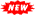##Book of DanielTony Garland

#### Journey verse-by-verse through this important book of the Old Testament. Knowledge of the Book of Daniel is foundational for understanding prophetic themes found elsewhere in Scripture, but especially in the Book of Revelation. Taught in Fall, 2008. [122 slides, 6 hours 47 minutes]

SessionDescription
00 ResourcesStudy Resources
We discuss study resources available to students. [8 slides, 17 minutes]
01 IntroductionIntroduction: Part 1
The introduction sets the stage for the verse-by-verse study to follow. A variety of preliminary subjects are discussed. In the first session of the introduction we discuss the goals of the course, attacks upon the Book of Daniel, and guidelines for study. [19 slides, 66 minutes]
02 IntroductionIntroduction: Part 2
The introduction sets the stage for the verse-by-verse study to follow. A variety of preliminary subjects are discussed. In the second session of the introduction we discuss the authorship of the Book of Daniel. [15 slides, 43 minutes]
03 IntroductionIntroduction: Part 3
The introduction sets the stage for the verse-by-verse study to follow. A variety of preliminary subjects are discussed. In the third session of the introduction we discuss the date of the Book of Daniel. [16 slides, 42 minutes]
04 IntroductionIntroduction: Part 4
The introduction sets the stage for the verse-by-verse study to follow. A variety of preliminary subjects are discussed. In the fourth session of the introduction we discuss the languages of the Book of Daniel. [14 slides, 33 minutes]
05 IntroductionIntroduction: Part 5
The introduction sets the stage for the verse-by-verse study to follow. A variety of preliminary subjects are discussed. In the fifth session of the introduction we discuss the versions and themes of the Book of Daniel. [15 slides, 49 minutes]
06 IntroductionIntroduction: Part 6
The introduction sets the stage for the verse-by-verse study to follow. A variety of preliminary subjects are discussed. In the sixth session of the introduction we discuss the structure of the Book of Daniel. [13 slides, 64 minutes]
07 IntroductionIntroduction: Part 7
The introduction sets the stage for the verse-by-verse study to follow. A variety of preliminary subjects are discussed. In the seventh session of the introduction we discuss the historical setting of the Book of Daniel. [22 slides, 93 minutes]

Copyright © 2008 by Tony Garland
(Version 0.8 generated on Sat Oct 4 14:37:21 2008)
contact@SpiritAndTruth.org